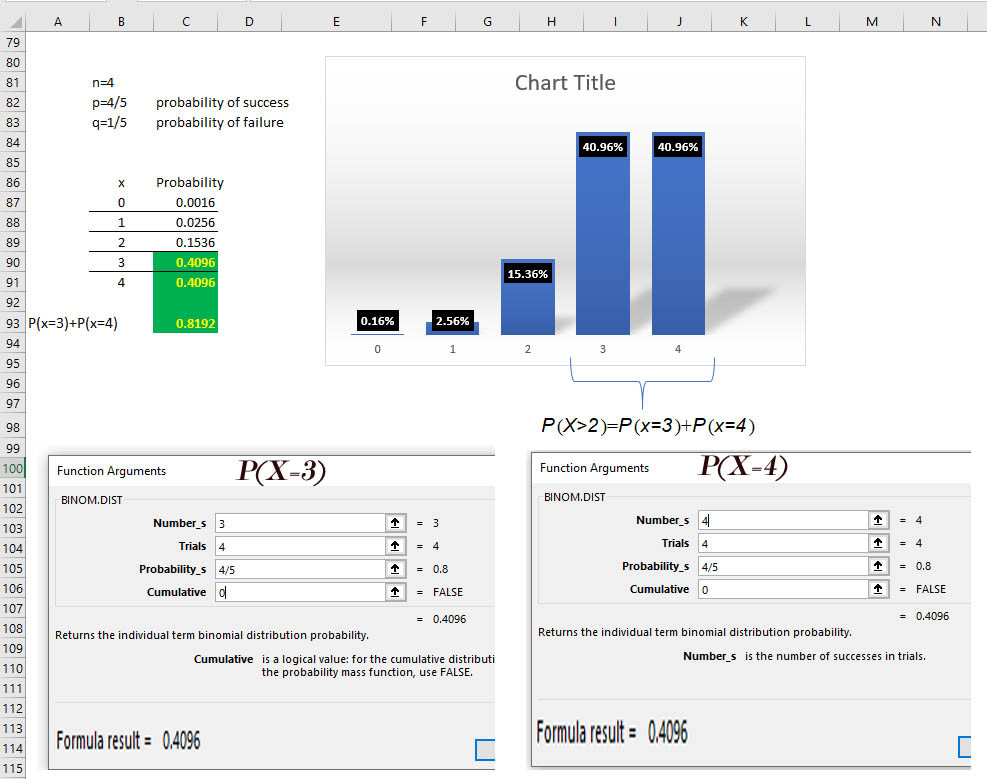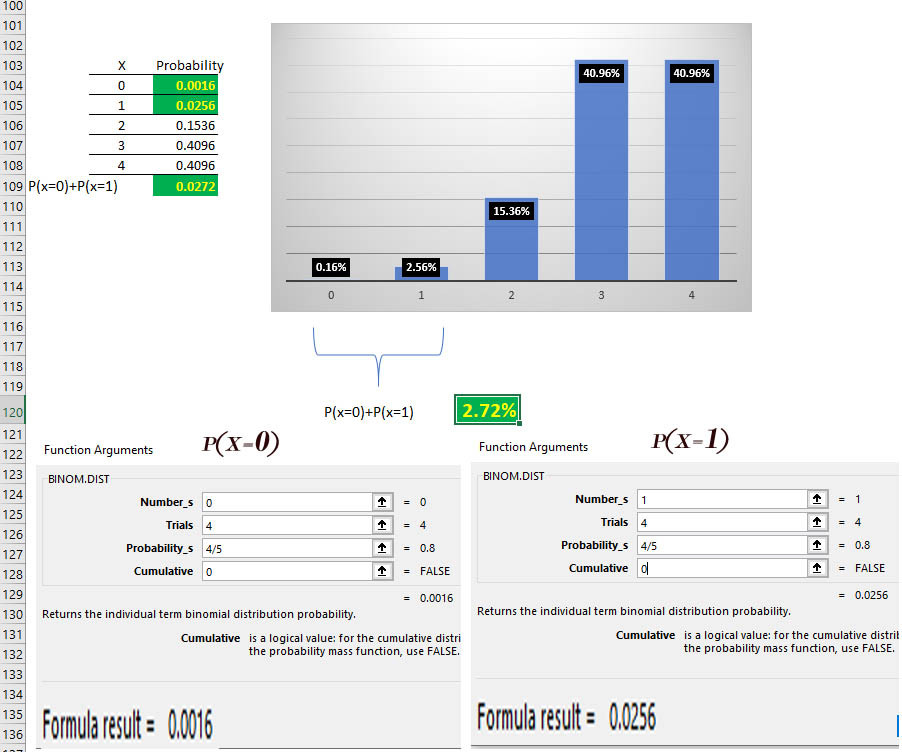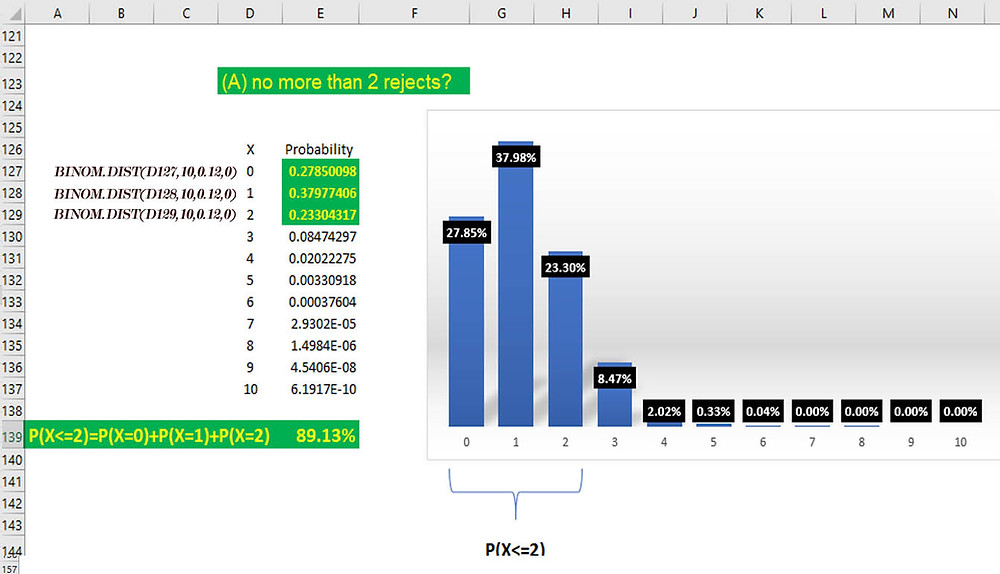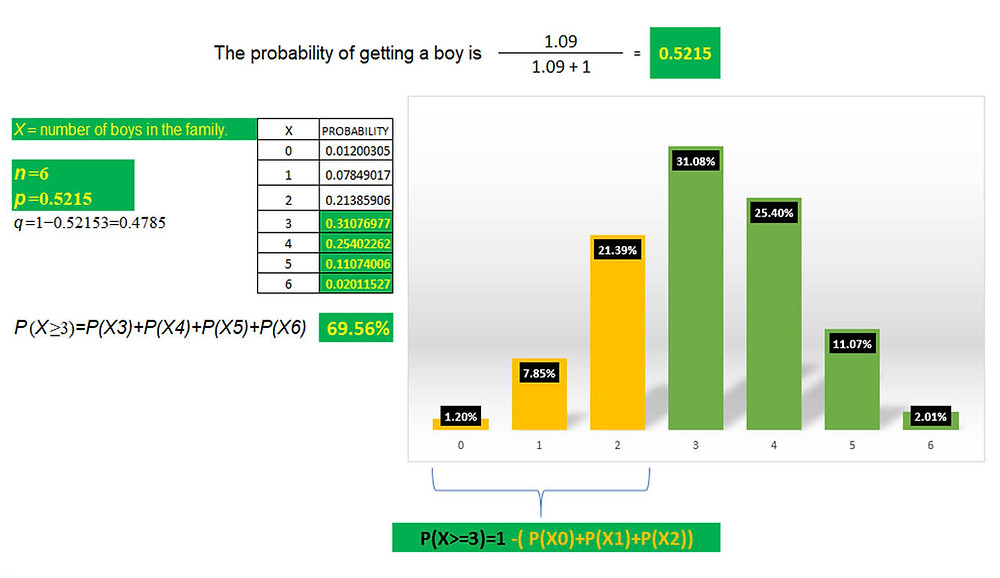Search
• Marek Vavrovic

# The Binomial Probability Distribution

A binomial experiment is one that possesses the following properties:

1. The experiment consists of n repeated trials;

2. Each trial results in an outcome that may be classified as a success or a failure (hence the name, binomial);

3. The probability of a success, denoted by p, remains constant from trial to trial and repeated trials are independent.

The number of successes X in n trials of a binomial experiment is called a binomial random variable.

The probability distribution of the random variable X is called a binomial distribution.

n = the number of trials

x = 0, 1, 2, ... n [sample space]

p = the probability of success in a single trial

q = the probability of failure in a single trial

q= 1 −p

## Mean and Variance of Binomial Distribution

If p is the probability of success and q is the probability of failure in a binomial trial, then the expected number of successes in n trials (i.e. the mean value of the binomial distribution) is

E(X) = μ = np

The variance of the binomial distribution is

V(X) = σ2 = npq

In a binomial distribution, only 2 parameters, namely n and p, are needed to determine the probability.

EXAMPLES

A die is tossed 3 times. What is the probability of no fives turning up?A die is tossed 3 times. What is the probability of 1 five turning up?A die is tossed 3 times. What is the probability of 3 fives ?Hospital records show that of patients suffering from a certain disease, 75% die of it. What is the probability that of 6 randomly selected patients, 4 will recover?

This is a binomial distribution because there are only 2 outcomes (the patient dies, or does not).

X =number who recover

n = 6 ; x=4

p= 0.25 probability of success

q=0.75 probability of failure

Let's make a table and graph first.Insert a Scatter Chart, then change the siries type to Bar ChartHospital records show that of patients suffering from a certain disease, 75% die of it. What is the probability that of 6 randomly selected patients, 4 will recover?

P(x=4)=3.29%A Japanese archer finds that on the average he hits the middle of a target 4 times out of 5. If he fires 4 shots, what is the probability of

(A) more than 2 hits?

n= 4 p=4/5

P(X>2)=P(x=3​)+P(x=4​)(B) at least 3 misses?

3 misses =1 hit

4 misses = 0 hits.A manufacturer of metal pistons finds that on the average, 12% of his pistons are rejected because they are either oversize or undersize. What is the probability that a batch of 10 pistons will contain

(A) no more than 2 rejects?

In this case, "success" means rejection.

n=10 p=0.12

P(X<=2)=P(X=0)+P(X=1)+P(X=2)(B) at least 2 rejects?

SOLUTION 1:SOLUTION 2:

=1−P(X≤1)

=1−(P(x0​)+P(x1​))

=1−(0.2785+0.37977)

=0.34173The ratio of boys to girls at birth in Singapore is at 1.09 : 1.

What proportion of Singapore families with exactly 6 children will have at least 3 boys? (Ignore the probability of multiple births.)A manufacture is making a product with 20% defect rate. If we select 5 items at the end of the assembly line, what is the probability of having 1 defected item?

C(5,1)=5 WE GOT 5 DIFFRENCE WAYS HOW TO DO THAT.## Recent Posts

See All

#### Index

Information functions Filter functions Table manipulation functions Grouping and summarizing Time intelligence functions OPENINGBALANCEYEAR OPENINGBALANCEYEAR(<Expression>,<Dates> [,<Filter>][,<YearEn